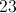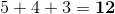# SSAT Elementary Level Math : How to find the perimeter of a triangle

## Example Questions

← Previous 1 3 4

### Example Question #1 : How To Find The Perimeter Of A Triangle

If one side of an equilateral triangle is 6, what is the perimeter of the triangle?Explanation:

To find the perimeter of any triangle you will need to add up all the sides.  For this triangle, you will add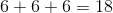since an equilateral triangle has 3 congruent sides.

### Example Question #1 : How To Find The Perimeter Of A Triangle

The perimeter of an equilateral triangle is 18 cm. Which choice shows the correct side lengths of the triangle?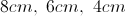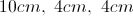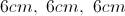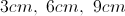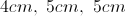Explanation:

When finding the perimeter of any triangle, you will need to add all three sides. In this case, it is important to note that this is the perimeter of an equilateral triangle. An equilateral triangle has three equal sides. All the choices add up to 18cm, but only one choice shows three equal sides.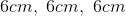### Example Question #1 : How To Find The Perimeter Of A Triangle

An equilateral triangle has a side of length 10. What is the perimeter of the triangle?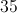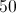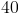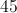Explanation:

In an equilateral triangle, all sides are of equal length. The perimeter of a triangle is the sum of the lengths of the three sides. Our equilateral has a side of length 10, so all sides will be equal to 10. We can find the perimeter by adding up 10 three times.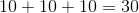### Example Question #2 : How To Find The Perimeter Of A Triangle

The perimeter of a scalene triangle is 21 mm.  Which choice shows the correct side lengths?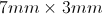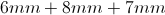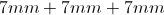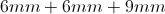Explanation:

When finding the perimeter of any triangle, you will need to add all three sides. In this case, it is important to note that this is the perimeter of a scalene triangle. A scalene triangle has no congruent/equal sides.  The only choice that adds up to 21 mm with no equal sides is: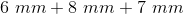### Example Question #1 : How To Find The Perimeter Of A Triangle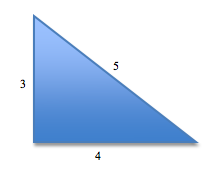What is the perimeter of the above triangle?

12

30

7.5

11

28

12

Explanation:

To find the perimeter of a triangle, add all of its three sides. 5 + 4 + 3 = 12

### Example Question #4 : How To Find The Perimeter Of A TriangleIf the blue triangle has three equal sides, what is the triangle's perimeter?Explanation:

Since the problem asks about an equilateral triangle (one that has three equal sides), we just need to multiply the given side's length by 3.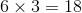### Example Question #2 : How To Find The Perimeter Of A Triangle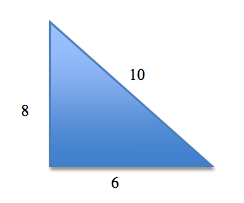What is the perimeter of the above triangle?

16

48

24

32

28

24

Explanation:

To find the perimeter of a triangle, simply add all three sides together.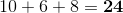### Example Question #5781 : Ssat Elementary Level Quantitative (Math)

A triangle has a perimeter ofinches. If all its sides are the same length, what is the length of one of its sides?inches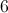inchesinchesinches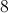inchesinches

Explanation:

A triangle hassides, and its perimeter is the length of all the sides added together. Since all the sides are the same length, dividing the perimeter bywill give the length of one side.divided byisinches.

### Example Question #6 : How To Find The Perimeter Of A Triangle

You can find the perimeter of a triangle if you know __________.

the height and base

three side lengths

its angles

the area

three side lengths

Explanation: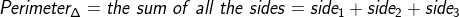### Example Question #7 : How To Find The Perimeter Of A Triangle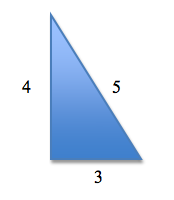What is the perimeter of the triangle?